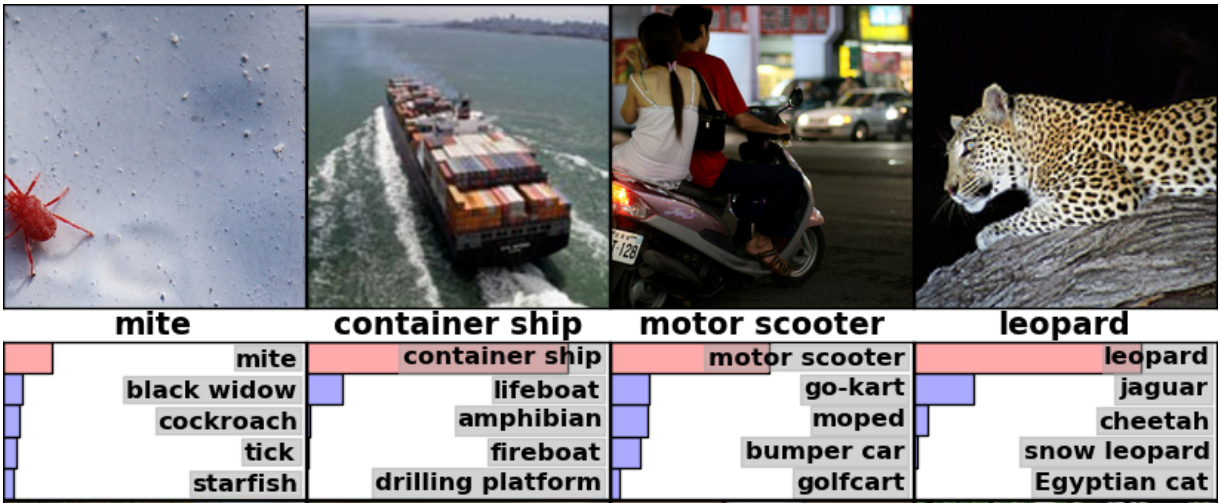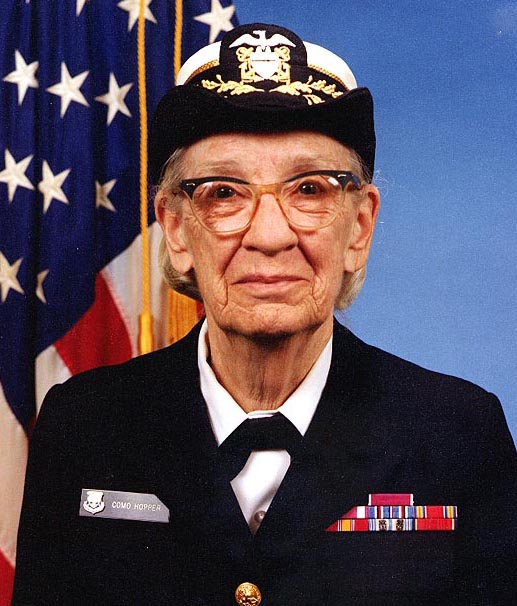# 图像识别

Inception-v3 从 2012 年就开始使用数据针对 ImageNet 这个大型视觉挑战任务训练了。将所有的图片分成像『斑马』，『达尔马西亚狗』，『洗碗工』等 [1000 个类别] 是计算机视觉领域的一个标准任务，例如下面这些图片就是 AlexNet 模型分类的结果：## Python API 的使用

`classify_image.py` 这个程序在第一次运行的时候会从 `tensorflow.org` 上下载训练好的模型。你需要保证你的硬盘有 200M 的可用空间。

``````cd models/tutorials/image/imagenet
python classify_image.py
````````````giant panda, panda, panda bear, coon bear, Ailuropoda melanoleuca (score = 0.88493)
indri, indris, Indri indri, Indri brevicaudatus (score = 0.00878)
lesser panda, red panda, panda, bear cat, cat bear, Ailurus fulgens (score = 0.00317)
custard apple (score = 0.00149)
earthstar (score = 0.00127)
``````

## C++ API 的使用

``````curl -L "https://storage.googleapis.com/download.tensorflow.org/models/inception_v3_2016_08_28_frozen.pb.tar.gz" |
tar -C tensorflow/examples/label_image/data -xz
``````

``````bazel build tensorflow/examples/label_image/...
``````

``````bazel-bin/tensorflow/examples/label_image/label_image
``````

``````I tensorflow/examples/label_image/main.cc:206] military uniform (653): 0.834306
I tensorflow/examples/label_image/main.cc:206] mortarboard (668): 0.0218692
I tensorflow/examples/label_image/main.cc:206] academic gown (401): 0.0103579
I tensorflow/examples/label_image/main.cc:206] pickelhaube (716): 0.00800814
I tensorflow/examples/label_image/main.cc:206] bulletproof vest (466): 0.00535088
````````````bazel-bin/tensorflow/examples/label_image/label_image --image=my_image.png
``````

`input_mean` from each pixel value, then divide it by `input_std`.

``````// 给定一张图片的文件名，读取它的数据，接着按照图片来解码，
// 缩放成我们需要的大小，然后按比例转换成我们想要的值。
Status ReadTensorFromImageFile(string file_name, const int input_height,
const int input_width, const float input_mean,
const float input_std,
std::vector<Tensor>* out_tensors) {
tensorflow::GraphDefBuilder b;
``````

``````  string input_name = "file_reader";
string output_name = "normalized";
b.opts().WithName(input_name));
``````

``````  // 现在让我们试着搞清楚它的文件类型，并解码。
const int wanted_channels = 3;
if (tensorflow::StringPiece(file_name).ends_with(".png")) {
} else {
// 如果它不是 PNG，那么就一定是 JPEG 了。
}
// 现在将图片数据转换成浮点型，这样我们就可以正常的计算它了。
tensorflow::Node* float_caster = tensorflow::ops::Cast(
// 在 TensorFlow 中图片操作的惯例就是所有的图片都是批量操作的，
// 所以它们是由 [batch, height, width, channel] 组成的 4 维数组。
// 因为我们只有一张图片，所以我们
// 必须一个 1 的 batch 维度，这样才能使用 ExpandDims()。
tensorflow::Node* dims_expander = tensorflow::ops::ExpandDims(
float_caster, tensorflow::ops::Const(0, b.opts()), b.opts());
// 双向调整，将我图片变成我们需要的维度。
tensorflow::Node* resized = tensorflow::ops::ResizeBilinear(
dims_expander, tensorflow::ops::Const({input_height, input_width},
b.opts().WithName("size")),
b.opts());
// 减去平均值并除以缩放的比例
tensorflow::ops::Div(
tensorflow::ops::Sub(
resized, tensorflow::ops::Const({input_mean}, b.opts()), b.opts()),
tensorflow::ops::Const({input_std}, b.opts()),
b.opts().WithName(output_name));
``````

``````  // 这里执行了我们刚构造的 GraphDef 的网络定义，
// 然后会在输出的 tensor 中返回一个结果。
tensorflow::GraphDef graph;
TF_RETURN_IF_ERROR(b.ToGraphDef(&graph));
``````

``````  std::unique_ptr<tensorflow::Session> session(
tensorflow::NewSession(tensorflow::SessionOptions()));
TF_RETURN_IF_ERROR(session->Create(graph));
TF_RETURN_IF_ERROR(session->Run({}, {output_name}, {}, out_tensors));
return Status::OK();
``````

`tf.Session`

``````// 从硬盘读取一个模型 graph 的定义，
// 创建一个你可以使用的 session 对象来运行它。
std::unique_ptr<tensorflow::Session>* session) {
tensorflow::GraphDef graph_def;
return tensorflow::errors::NotFound("Failed to load compute graph at '",
graph_file_name, "'");
}
``````

``````  session->reset(tensorflow::NewSession(tensorflow::SessionOptions()));
Status session_create_status = (*session)->Create(graph_def);
if (!session_create_status.ok()) {
return session_create_status;
}
return Status::OK();
}
``````

`GetTopLabels()` 这个函数和图片载入的函数很像，在这种情况下，我们想要获得运行主 graph 的结果，并且把它转化成一个有最高分标签的有序列表。就像图片加载器一样，它创建了一个 `GraphDefBuilder`，添加了一些节点，并且运行了一个短的 graph 来获得一对 tensors 的输出。在这种情况下他们代表着有序的得分以及最高分结果的下标位置。

``````// 分析 Inception graph 的输出信息，并且在它们相关的分类上获取
// 最高的得分以及在 tensor 中的位置。
Status GetTopLabels(const std::vector<Tensor>& outputs, int how_many_labels,
Tensor* indices, Tensor* scores) {
tensorflow::GraphDefBuilder b;
string output_name = "top_k";
tensorflow::ops::TopK(tensorflow::ops::Const(outputs, b.opts()),
how_many_labels, b.opts().WithName(output_name));
// 这里执行了我们刚构造的 GraphDef 的网络定义，
// 然后会在输出的 tensor 中返回一个结果。

tensorflow::GraphDef graph;
TF_RETURN_IF_ERROR(b.ToGraphDef(&graph));
std::unique_ptr<tensorflow::Session> session(
tensorflow::NewSession(tensorflow::SessionOptions()));
TF_RETURN_IF_ERROR(session->Create(graph));
// TopK 这个节点返回了两个输出，得分和他们的原始下标，
// 所以我们需要添加 :0 和 :1 来区分它们。
std::vector<Tensor> out_tensors;
TF_RETURN_IF_ERROR(session->Run({}, {output_name + ":0", output_name + ":1"},
{}, &out_tensors));
*scores = out_tensors;
*indices = out_tensors;
return Status::OK();
``````

`PrintTopLabels()` 这个函数获取了那些有序的结果，然后把它们友好的打印了出来。`CheckTopLabel()` 这个函数也是老熟人了，但是为了调试，我们还是要确定下最顶部的标签就是我们最想要的那个。

``````int main(int argc, char* argv[]) {
// 我们需要调用这个函数来设置 TensorFlow 的全局状态。
tensorflow::port::InitMain(argv, &argc, &argv);
Status s = tensorflow::ParseCommandLineFlags(&argc, argv);
if (!s.ok()) {
LOG(ERROR) << "Error parsing command line flags: " << s.ToString();
return -1;
}

// 首先我们加载并初始化模型。
std::unique_ptr<tensorflow::Session> session;
string graph_path = tensorflow::io::JoinPath(FLAGS_root_dir, FLAGS_graph);
return -1;
}
``````

``````  // 从硬盘中获取图片并转换成浮点数组，
// 调整大小并标准化成主 graph 要求的格式。
std::vector<Tensor> resized_tensors;
string image_path = tensorflow::io::JoinPath(FLAGS_root_dir, FLAGS_image);
image_path, FLAGS_input_height, FLAGS_input_width, FLAGS_input_mean,
FLAGS_input_std, &resized_tensors);
return -1;
}
const Tensor& resized_tensor = resized_tensors;
``````

``````  // 真正的通过模型执行图片
std::vector<Tensor> outputs;
Status run_status = session->Run({{FLAGS_input_layer, resized_tensor}},
{FLAGS_output_layer}, {}, &outputs);
if (!run_status.ok()) {
LOG(ERROR) << "Running model failed: " << run_status;
return -1;
}
``````

``````  // 这里是为了保证我们在默认设置下得到了我们想要结果的自动测试程序。
// 我们知道标签 866（军装）应该
if (FLAGS_self_test) {
bool expected_matches;
Status check_status = CheckTopLabel(outputs, 866, &expected_matches);
if (!check_status.ok()) {
LOG(ERROR) << "Running check failed: " << check_status;
return -1;
}
if (!expected_matches) {
LOG(ERROR) << "Self-test failed!";
return -1;
}
}
``````

``````  // 用我们生成的结果做一些有趣的事情。
Status print_status = PrintTopLabels(outputs, FLAGS_labels);
``````

``````  if (!print_status.ok()) {
LOG(ERROR) << "Running print failed: " << print_status;
return -1;
}
``````

## 进一步学习的资源

TF Layers 教程：构建卷积神经网络